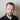# Example Bitcoin seasonality strategy with Pandas

March 17, 2020 | bitcoin

OK, my plan for this strategy is as follows:

• First, we’ll split Bitcoin returns into weekdays,
• then we’ll rate those returns using Sharpe ratio of in sample data,
• and then we’ll sum up 3 best weekdays for final returns.

Pretty simple. I hope this will demonstrate in a way how quantitative strategies are created.

## Split by weekday and find best 3

from numpy import sqrt

def get_week_returns(df, day):
"""
Get returns for a weekday. 0 is Monday.
"""
df = df[df.index.weekday==day]
df['pct'] = rm_inf(df, 'pct')
return df['pct']

def week_seasonality(df):
"""
Concatenate all weekdays into one DataFrame
"""
df = df.dropna()
d = concat([get_week_returns(df, 0), get_week_returns(df, 1), get_week_returns(df, 2), get_week_returns(df, 3), get_week_returns(df, 4), get_week_returns(df, 5), get_week_returns(df, 6)], axis=1)
d.columns = ['1', '2', '3', '4', '5', '6', '7']
d = d.fillna(0.0)
return d

def get_sharpe(returns):
"""
Calculate Sharpe ratio.
"""
return returns.mean() / returns.std() * sqrt(252)

def week_sharpe(df):
"""
Print all Sharpe ratios.
"""
by_weekdays = week_seasonality(df)
for i in range(1, 8):
s = get_sharpe(by_weekdays['{}'.format(i)])
print(s)

if __name__ == '__main__':
# get Bitcoin daily returns
df = get_btc()
df['pct'] = df['close'].pct_change()

# split into in sample and out of sample 50/50
half = int(len(df.index) / 2)
insample = df.iloc[0:half]
outsample = df.iloc[half:]

# get Sharpe ratios
week_sharpe(insample)

Our transformations got us to the following DataFrame split into Bitcoin returns by weekday:

              close    high     low     open       pct
Date
2017-05-09  1760.00  1815.0  1695.1  1703.50  0.033167
2017-05-10  1796.90  1817.4  1722.3  1760.00  0.020966
2017-05-11  1853.90  1908.1  1763.6  1796.90  0.031721
2017-05-12  1735.00  1864.8  1700.2  1853.90 -0.064135
2017-05-13  1819.50  1825.0  1666.0  1735.00  0.048703
2017-05-14  1827.30  1852.4  1775.2  1819.50  0.00428

After running in sample Sharpe ratios, we got following results

1.7428274061924838
1.1448755337608776
-0.6395336247038247
1.2334486785016472
0.2753996463863417
0.8361228207768844
0.44588909750430494

We can write automatic sorting of those ratios in the real trading, but for sake of simplicity, I’ll use manual selection here.

As we can see, Monday, Tuesday and Thursday are best for given data. We’ll use those days for our out of sample returns calculation.

To avoid look-ahead bias, it is important to use in and out of sample splits. I’ve already ran those tests for all data and it shows that best days are Monday, Friday and Saturday, 66% different than in our in sample data. If we would choose all data rating instead of in-sample data, we would introduce look-ahead bias, which will show better returns for an in-sample period, but unlikely to perform in the future (i.e., out of sample).

We know that seasonality changed from in-sample to out of sample data, so how can we improve this strategy in the real trading? Probably, we should run something like rolling Sharpe ratio and dynamically change days according to changing performance.

OK, here are the results for all data using in-sample sorting:

from matplotlib import pyplot as plt

outsample = outsample.dropna()
outsample['1'] = get_week_returns(outsample, 0)
outsample['2'] = get_week_returns(outsample, 1)
outsample['3'] = get_week_returns(outsample, 3)
outsample = outsample.fillna(0.0)
outsample['returns'] = outsample['1'] + outsample['2'] + outsample['3']
outsample['cumulative'] = outsample['returns'].cumsum()
outsample['cumulative'].plot()
plt.show()Not so good as you see. It happens because trading conditions are always changing.

Sharpe ratio of out of sample data is -0.0075, at break-even.

As this is always changing, we can probably improve it using rolling Sharpe ratio.

With rolling windows calculations will become increasingly complex, so to make things faster here, we’ll use only two days - Monday and Saturday. For all days, this should be completely rewriten.

# get Monday
w1 = get_week_returns(df, 0)
w1 = w1.to_frame()
w1.columns = ['1']
w1['1-sharpe'] = w1['1'].rolling(window=30).mean() / w1['1'].rolling(window=30).std() * sqrt(252)

# Get Saturday
w2 = get_week_returns(df, 5)
w2 = w2.to_frame()
w2.columns = ['2']
w2['2-sharpe'] = w2['2'].rolling(window=30).mean() / w2['2'].rolling(window=30).std() * sqrt(252)

# Concatenate and fill NaNs with zeros
d = concat([w1, w2], axis=1)
d = d.fillna(0.0)

# If Sharpe ratio of Monday is greater than Sharpe ratio of Saturday, buy Monday
# We shift Saturday to future by 2 days to be able to do those comparisons
d['returns'] = where(d['1-sharpe'] > d['2-sharpe'].shift(2), d['1'], 0)
# And if Saturday Sharpe ratio is greater than Sharpe ratio of Monday, we buy Saturday
d['returns'] += where(d['1-sharpe'] < d['2-sharpe'].shift(), d['2'], 0)
# Get cumulative returns and plot results
d['cumulative'] = d['returns'].cumsum()
d['cumulative'].plot()
plt.show()

We don’t need in sample/ out of sample splits here, rolling windows do that automatically.It looks much better and has Sharpe ratio of 1.749.

For better comparison, we should probably use same data as in our initial strategy. When running on out of sample data, I got Sharpe ratio of 1.24, still much better than just using simple data split. And this is just trading 2 days a week!Written by Tadas Talaikis